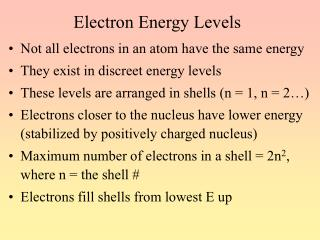DownloadDownload PresentationElectron Energy Levels

# Electron Energy Levels

Download Presentation## Electron Energy Levels

- - - - - - - - - - - - - - - - - - - - - - - - - - - E N D - - - - - - - - - - - - - - - - - - - - - - - - - - -
##### Presentation Transcript

1. Electron Energy Levels • Not all electrons in an atom have the same energy • They exist in discreet energy levels • These levels are arranged in shells (n = 1, n = 2…) • Electrons closer to the nucleus have lower energy (stabilized by positively charged nucleus) • Maximum number of electrons in a shell = 2n2, where n = the shell # • Electrons fill shells from lowest E up

2. Electron Shells

3. Electromagnetic Radiation • Electromagnetic radiation consists of photons (particles) that travel as waves • Examples: light, x-rays and radio waves • Distance between peaks is called the wavelength • Longer wavelength = lower energy • Electrons can jump to a higher energy level by absorbing energy (light, heat…) • When they drop back down to their original E, sometimes visible light is emitted (neon lights)

4. Electromagnetic Spectrum

5. Subshells • Electrons within each shell are not all the same E • They are arranged into subshells (s, p, d, f) • The E order of the subshells goes s < p < d < f • Number of subshells = shell number • n = 1 has only s, n = 2 has s and p, etc. • As n increases, main E levels are closer, so there is some overlap (4s subshell is lower E than 3d)

6. Orbitals • Each subshell is made up of orbitals • An orbital is a region of space in which electrons of that E level are most likely to be found • The s subshell has only one orbital (spherical) • The p subshell has three orbitals (dumbell shaped) • Each orbital can contain 0, 1 or 2 electrons • So s has a max of 2 electrons and p has a max of 6 electrons (2 for each orbital)

7. Electron Configurations • The electron configuration shows how the electrons are arranged in the subshells of an atom • Written as the shell number and subshell symbol, with number of electrons in the subshell as superscript • Examples: Li = 1s22s1 S = 1s22s22p63s23p4 • Use periodic table to get electron configuration • (check that superscripts add up total number of electrons = atomic number)